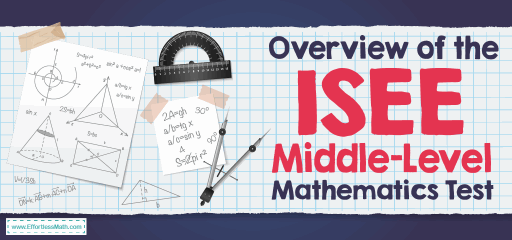# Overview of the ISEE Middle-Level Mathematics TestThe Independent School Entrance Exam is as known as ISEE for grades 7 and 8 is an entrance examination used by independent schools across the country to identify the potential student’s strengths in all of the core academic skills needed to succeed in educational goals.

The ISEE Middle Level consists of a total of 160 multiple-choice questions and 2 hours, 40 minutes, divided into four sections, each of which examines different skills. Also, there is a fifth section in which applicants must prepare an essay. The five sections involve:

• Verbal Reasoning: 40 Questions – 20 minutes
• Quantitative Reasoning: 37 Questions – 35 minutes
• Reading Comprehension: 36 Questions – 35 minutes
• Mathematics Achievement: 47 Questions – 40 minutes
• Essay: 1 Prompt – 30 minutes

The ISEE Middle-Level mathematics involves two parts: Quantitative Reasoning and Mathematics Achievement. Here there is some information about ISEE Middle-Level Mathematics.

## How is the structure of the Quantitative Reasoning of the ISEE Middle-Level Test?

The Quantitative Reasoning section of ISEE assesses the development of your reasoning skills. This section, unlike the amount of math you have learned, evaluates how you think mathematically. In the ISEE quantitative reasoning section, calculations are not necessary, because the importance of this section is your ability to reason and think mathematically.

There are two different types of questions on the quantitative reasoning section of ISEE Middle-Level: Word Problems and Quantitative Comparison questions.

The Word Problem questions, unlike mathematical notation, provide background information in the form of a text. The question is then followed by four answer options, one of which is the correct answer.

The Quantitative Comparison questions found only at middle and upper levels, offer two values for comparison. There are two columns, column A and column B, showing comparable quantities. The answer options among the Quantitative Comparison questions, four options are always the same:

A. The quantity in Column A is greater.

B. The quantity in Column B is greater.

C. The two quantities are equal.

D. The relationship can’t be determined from the information given.

## How is the structure of the Mathematics Achievement of the ISEE Middle-Level Test?

The Mathematics Achievement section is designed to test knowledge of mathematical processes and terms learned during the school years. Unlike the quantitative reasoning section, this section forces students to do actual calculations and use math skills.

This section contains 47 questions from various math topics:

### Numbers and Operations

• Analyze, perform operations on, and compare integers, decimals, fractions, and percent

### Algebra

• Represent, interpret, and evaluate math situations involving functions, patterns, and relationships

### Geometry

• Understand properties and characteristics of geometric figures
• Describe geometric shapes

### Measurement

• Know and use formulas for measurable attributes of objects

### Data Analysis & Probability

• Collect, interpret, display, and make predictions about a set of data
• Apply and understand the basic concepts of probability

This section requires students to perform calculations to solve problems. Scratch paper and calculator are not allowed in the test. The students are allowed to write in the test booklet.

## Do you give a formula sheet on theISEE Middle-Level Mathematics Test?

You will not be given formula in the ISEE Middle-Level Math Test. You must remember all the important formulas.

## Is the ISEE Middle-Level Test hard?

The ISEE Middle-Level test is difficult, but with enough study and constant practice, you can succeed.

## Can you use a calculator on the ISEE Middle-Level Mathematics Test?

The students are not allowed to use the calculator on the ISEE Middle-Level test.

## How is the ISEE Middle-Level Mathematics Test?

The ISEE Middle-Level scaled scores for mathematics from 760 to 940. The scaled score is derived from the raw score, which is the number of questions the student answered correctly. Here is more information about the ISEE Middle-Level scoring system.

## The Best Books to Ace the ISEE Middle-Level MathTest

### What people say about "Overview of the ISEE Middle-Level Mathematics Test - Effortless Math: We Help Students Learn to LOVE Mathematics"?

No one replied yet.

X
23% OFF

Limited time only!

Save Over 23%

SAVE \$5

It was \$21.99 now it is \$16.99